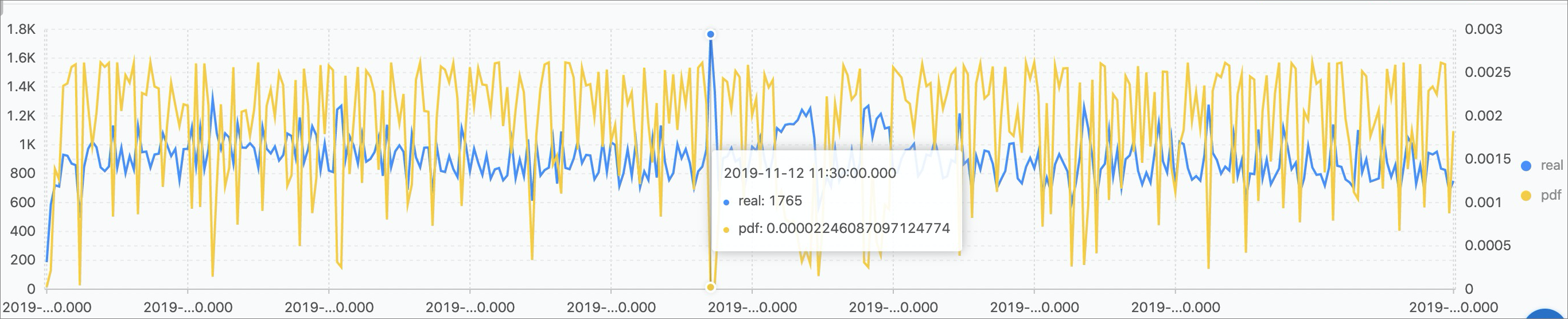Kernel density estimation is a non-parametric test method. It is used to estimate unkonwn density functions in probability theory.

Kernel density estimation functions use a smooth peak function to simulate the real probability distribution curve by fitting the observed data points.
• Function format:
``select kernel_density_estimation(bigint stamp, double value, varchar kernelType)``
• Parameters
Parameter Description
stamp Unix timestamp. Unit: second.
value Observed value.
kernelType
• box: rectangle.
• epanechnikov: Epanechnikov curve.
• gaussian: Gaussian curve.
• Output result
Display item Description
unixtime The time of the source data.
real Observed value.
pdf The probability of each point.
• Examples
• Sample code:
``````* |
select
date_trunc('second', cast(t1 as bigint)) as time, t1 as real, t1 as pdf from (
select kernel_density_estimation(time, num, 'gaussian') as res from (
select __time__ - __time__ % 10 as time, COUNT(*) * 1.0 as num from log group by time order by time)
), unnest(res) as t(t1)  limit 1000``````
• Sample result: